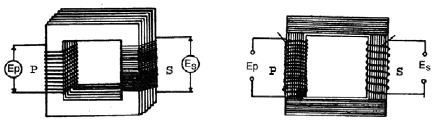Physics

# What is Construction?

A transformer is made on a soft iron core in the rectangular form in which two coils of insulated copper wire are inserted in its two opposite limbs (below Fig) The coil in which an a.c. or emf is applied is known as the primary coil. The coil in which an a.c or emf is induced is known as the secondary coil. In the step-up transformer, the number of turns in the secondary coil is greater than that in the primary coil. In step down transformer, the number of turns in the secondary is less than that in the primary coil.Let an a.c emf EP be applied to a primary coil nP turns. A current IP flows in this coil. This curent by magnetizing the core produces magnetic lines of force that ultimately produce induced emf in the primary coil. If there is no diminution of the magnetic lines same number of magnetic lines remain associated with each turn of the secondary coil. Consequently, an emf is induced in the secondary coil.

If the number of turns in the secondary coil be nS and the induced emf be ES,

We have: EP/ ES = nP/ nS

That is to say that the induced emf in the coil is proportional to the number of turns.

For a step up transformer nS > nP and for a step clown transformer nS < nP. If no energy is dissipated in the transformer core, the energy input to the primary coil is wholly transferred to the secondary coil.

Therefore, the voltage of the primary coil x current of primary coil = Voltage of secondary coil x current of secondary coil

i.c. EP IP = ES IS

Therefore, EP/ ES = IP/ IS

This equation suggests that the rate at which a transformer reduces the voltage is exactly equal to the rate at which it increase the current so that the total power remains constant. Therefore, a transformer transforms both the voltage and the current.

For transmission of electric power over long distances step up transformer is used. Step down transformer is used for domestic power supply such as radio, television, tape recorder, VCR, VCP, electric watch etc.